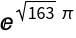#Function Repository Resource:

# IntegerChop

Round near-integer values to integers

Contributed by: Ed Pegg Jr
 ResourceFunction["IntegerChop"][n] rounds n to an integer if n is close to an integer. ResourceFunction["IntegerChop"][n,delta] rounds n if the absolute difference between n and the result is within delta.

## Details

The default tolerance for delta is 10-10.

## Examples

### Basic Examples (2)

Round a real number close to 7 to the integer 7:

 In:=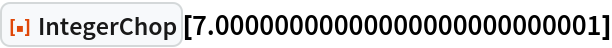Out=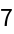The following values are not close enough and remain unchanged:

 In:=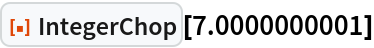Out=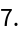In:=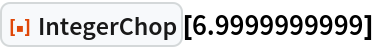Out=Round elements of a list that are close to integers:

 In:=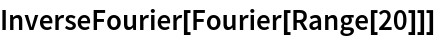Out=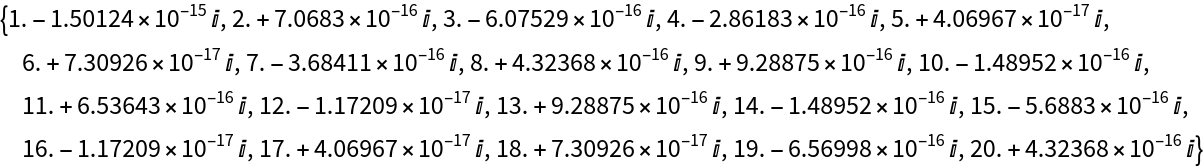In:=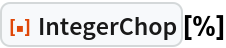Out=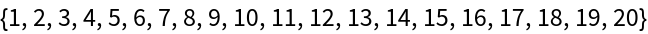### Scope (3)

The Ramanujan constant gets chopped by default:

 In:=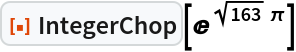Out=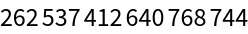The number is within 10-10 of an integer:

 In:=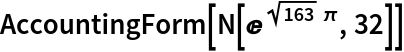Out=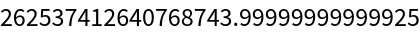A tighter bound eliminates the chopping:

 In:=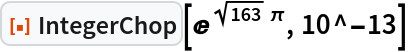Out=